Infinitely-distant elements

infinitely-remote elements, improper elements, ideal elements

Elements (points, straight lines, planes, etc.), generated by extending a given affine space to a compact space. Infinitely-distant elements are one of the forms in which the "actual" infinity is manifested in various mathematical theories. The continuous connection of the finite and the infinite is manifested by the fact that infinitely-distant elements are meaningful only in as far as they are considered in the context of some concrete compactification of a given "finite" space. The types of infinitely-distant elements resulting from the most frequent methods of compactification of finite-dimensional Euclidean spaces are described below.

1) If infinitely-distant elements (pointsand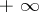) are introduced, the number axisis completed to the compact extended number axis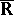, which is homeomorphic to a (closed) segment. Another way of compactification consists in imbeddingin the real projective straight line, which is homeomorphic to the circle(cf. Projective space);is then completed by a single, unique infinitely-distant point.

2) By the addition of a single, unique infinitely-distant pointthe finite complex planeis completed to the compact extended complex plane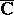, which is homeomorphic to the complex projective straight line or the Riemann sphere(the unit sphere in the Euclidean space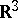).

3) By the addition of a single, unique infinitely-distant pointthe-dimensional real number space,, is completed to the compact extended number space, which is homeomorphic to the sphere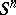; this homeomorphism can be demonstrated visually by stereographic projection. Another method of compactification consists in imbeddingin the-dimensional real projective space. If, these two compactifications are not homeomorphic.

For instance, to parallel straight lines, in the projective planethe same infinitely-distant point corresponds, while different infinitely-distant points correspond to non-parallel straight lines. All infinitely-distant points of the planeconstitute the infinitely-distant straight line. In a similar way, each plane in the projective spaceis completed by an infinitely-distant straight line. All infinitely-distant points and infinitely-distant straight lines in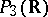constitute the infinitely-distant plane. In general, the infinitely-distant elements in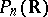of dimension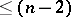constitute the-dimensional infinitely-distant hyperplane.

4) A compactification of the complex-dimensional number space,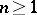, is also possible by imbeddingin the complex-dimensional projective space. In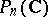, too, all infinitely-distant elements of dimensionconstitute the complex-dimensional infinitely-distant hyperplane. Another method of compactification consists in extendingto the extended complex space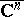, which is the topological product ofcopies of. If, the spacesandare not homeomorphic. The infinitely-distant points of the spaceare the pointsin which at least one coordinate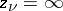. The set of all infinitely-distant points of the spaceis naturally subdivided intosets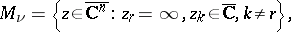each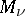having dimension. The point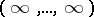belongs to all,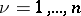. For real functions on, the one-point compactification (cf. Aleksandrov compactification)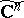, homeomorphic toor to the sphere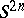, is also used.